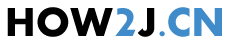5分3秒

## 封装类

package digit; public class TestNumber { public static void main(String[] args) { int i = 5; //把一个基本类型的变量,转换为Integer对象 Integer it = new Integer(i); //把一个Integer对象，转换为一个基本类型的int int i2 = it.intValue(); } }
```package digit;

public class TestNumber {

public static void main(String[] args) {
int i = 5;

//把一个基本类型的变量,转换为Integer对象
Integer it = new Integer(i);
//把一个Integer对象，转换为一个基本类型的int
int i2 = it.intValue();

}
}
```

## Number类

Byte,Short,Integer,Long,Float,Doublepackage digit; public class TestNumber { public static void main(String[] args) { int i = 5; Integer it = new Integer(i); //Integer是Number的子类，所以打印true System.out.println(it instanceof Number); } }
```package digit;

public class TestNumber {

public static void main(String[] args) {
int i = 5;

Integer it = new Integer(i);
//Integer是Number的子类，所以打印true
System.out.println(it instanceof Number);
}
}
```

## 基本类型转封装类

package digit; public class TestNumber { public static void main(String[] args) { int i = 5; //基本类型转换成封装类型 Integer it = new Integer(i); } }
```package digit;

public class TestNumber {

public static void main(String[] args) {
int i = 5;

//基本类型转换成封装类型
Integer it = new Integer(i);

}
}
```

## 封装类转基本类型

package digit; public class TestNumber { public static void main(String[] args) { int i = 5; //基本类型转换成封装类型 Integer it = new Integer(i); //封装类型转换成基本类型 int i2 = it.intValue(); } }
```package digit;

public class TestNumber {

public static void main(String[] args) {
int i = 5;

//基本类型转换成封装类型
Integer it = new Integer(i);

//封装类型转换成基本类型
int i2 = it.intValue();

}
}
```

## 自动装箱

package digit; public class TestNumber { public static void main(String[] args) { int i = 5; //基本类型转换成封装类型 Integer it = new Integer(i); //自动转换就叫装箱 Integer it2 = i; } }
```package digit;

public class TestNumber {

public static void main(String[] args) {
int i = 5;

//基本类型转换成封装类型
Integer it = new Integer(i);

//自动转换就叫装箱
Integer it2 = i;

}
}
```

## 自动拆箱

package digit; public class TestNumber { public static void main(String[] args) { int i = 5; Integer it = new Integer(i); //封装类型转换成基本类型 int i2 = it.intValue(); //自动转换就叫拆箱 int i3 = it; } }
```package digit;

public class TestNumber {

public static void main(String[] args) {
int i = 5;

Integer it = new Integer(i);

//封装类型转换成基本类型
int i2 = it.intValue();

//自动转换就叫拆箱
int i3 = it;

}
}
```

## int的最大值，最小值

int的最大值可以通过其对应的封装类Integer.MAX_VALUE获取
package digit; public class TestNumber { public static void main(String[] args) { //int的最大值 System.out.println(Integer.MAX_VALUE); //int的最小值 System.out.println(Integer.MIN_VALUE); } }
```package digit;

public class TestNumber {

public static void main(String[] args) {

//int的最大值
System.out.println(Integer.MAX_VALUE);
//int的最小值
System.out.println(Integer.MIN_VALUE);

}
}
```

## 练习-装箱拆箱Or姿势不对,事倍功半! 点击查看做练习的正确姿势
1. 对byte,short,float,double进行自动拆箱和自动装箱

2. byte和Integer之间能否进行自动拆箱和自动装箱

3. 通过Byte获取byte的最大值

## 答案-装箱拆箱2分11秒 本视频采用html5方式播放，如无法正常播放，请将浏览器升级至最新版本，推荐火狐，chrome，360浏览器 如果装有迅雷，播放视频呈现直接下载状态，请调整 迅雷系统设置-基本设置-启动-监视全部浏览器 (去掉这个选项)

package digit; public class TestNumber { public static void main(String[] args) { // 1. 对byte,short,float,double进行自动拆箱和自动装箱 byte b = 1; short s = 2; float f = 3.14f; double d = 6.18; // 自动装箱 Byte b1 = b; Short s1 = s; Float f1 = f; Double d1 = d; // 自动拆箱 b = b1; s = s1; f = f1; d = d1; // 2. byte和Integer之间能否进行自动拆箱和自动装箱 Integer i1 = b; //不能把byte直接自动装箱成Integer b = new Integer(1); //也不能把Integer自动拆箱成 byte // 3. 通过Byte获取byte的最大值 System.out.println(Byte.MAX_VALUE); } }

HOW2J公众号，关注后实时获知最新的教程和优惠活动，谢谢。2019-11-18 自动装、拆
 软件测试 关于 JAVA 基础-数字与字符串-装箱拆箱 的提问 int number = 5; // 把基本类型变量转换成Intger对象（封装类型） Integer integer = new Integer(5); // 把Integer（封装类）转换成一个基本类型int int number2 = integer.intValue(); ``` // byte自动装箱 byte byte1 = 32; Byte byte2 = byte1; System.out.println(byte2 instanceof Byte); // byte自动拆箱 byte byte3 = byte2; // System.out.println(byte3 instanceof Byte); System.out.println(Byte.MAX_VALUE); // byte最大值 // short short short1 = 13; Short short2 = short1; // 自动装箱 short short3 = short2; // 自动拆箱 // float float float1 = 3.3f; Float float2 = float1; // 自动装箱 float float3 = float2; //自动拆箱 // double double double1 = 0.16d; Double double2 = double1; // 自动装箱 double double3 = double2; // 自动拆箱 // int byteInt = 22; // Byte byteInt1 = byteInt; ```

2019-11-14 交作业
 万里山 关于 JAVA 基础-数字与字符串-装箱拆箱 的提问 int和byte不能自动装箱和拆箱，把那个byte强制转换成int然后就可以自动装箱拆箱了，这样算不算。>

1 个答案

2019-11-03 交作业1
2019-11-03 交作业
2019-10-24 交作业
2019-10-08 作业
2019-09-23 交作业
2019-09-15 小头答案
2019-08-27 装箱拆箱
2019-08-23 交作业-自动装箱和自动拆箱
2019-07-29 交作业
2019-05-29 关于byte和Integer之间是否可以自动装箱or拆箱以及我自己遇到的问题
2019-05-29 关于byte和Integer之间是否可以自动装箱or拆箱以及我自己遇到的问题
2019-05-17 写作业
2019-05-13 ZZ交作业 装箱拆箱
2019-04-22 交作业
2019-04-22 加油周一
2019-04-17 旋转跳跃
2019-04-10 编译器的时候没有报错为什么运行的时候有这个错误？
2019-03-06 交作业交作业~
2019-01-13 作业参考
2019-01-04 交作业
2018-12-10 装箱拆箱！
2018-11-21 装箱和拆箱 主要应用在什么地方呢？？？
2018-11-12 交作业
2018-09-17 交作业交作业交作业交作业交作业交作业交作业
2018-08-30 按在地上摩擦~
2018-08-29 int 可以吧Byte自动拆箱，其他都不行
2018-08-27 我们一起成长~
2018-05-13 交作业
2018-05-07 交作业
2018-03-07 提交作业啊.
2018-03-05 自动拆箱这个怎么解释
2017-12-12 经过后面的学习，我胡汉三回来写个小记
2017-12-04 作业提交
2017-10-11 只有把Byte 自动拆箱到int可以。
2016-11-01 可以直接把Byte拆箱到int耶
2016-05-12 直接输出Integer类型不行吗，为什么要转换为int类型呢